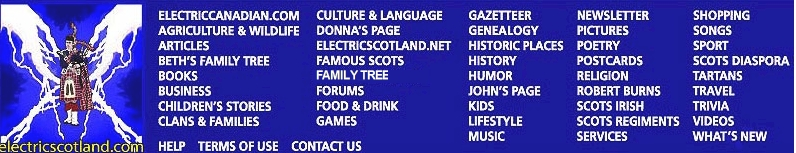Rolfin's Orb Book 7 - Amber Word Search Puzzle
 `H T N D H R H S H A R D O W M I L L J G M O V M M J C S R D ` `I T H A D X R Q Y Q F I G C A C C F S A E E K W J A M I E R ` `I T E C U A A X T F M R G N Y J Q Q S D X V Z J T K R P C V ` `S S Z N X E L I A M B J M L A K A G V E I E Q R N A A O Y F ` `G Y X E N K N R B D C T R Y N S X H B C C C I X S O L B N E ` `X O O J L E O F Y G Z D A Z E H Z H A T O O D W O Q Y S Q A ` `S B I C T S K F D Y R C M J V M L L G Q N V G O R A E F C Z ` `Q Y V J E P A M A U V L P D L A U M H A K C U D T U J W P Y ` `E T O N E C R V L M Z G I I Q X N B U S Z S T I D C S C H G ` `Q H N D T F U K V G A W T I O L J Z L X Z O S R Q A E A C W ` `B U A Y M W Y J R C Y J Z B Y R N E G X R O D Q N H N W B J ` `L L E Z D F V H S D L P E S O X C W N A R X E C G N Y G A Z ` `O Y L T G J C M X L A M L X T L M C O A Z H R J E Y P N S Z ` `J S R Q Z D N E P D I W L V A O E R G L K S A E P K Q X G H ` `Q B O L D I T C W W C X M B K P F D W I D L J N U U Z G M O ` `G X J T Q F T P Z H H G I P B H P U N G E D Q A S R Y G J I ` `J S X F K U F I C W P N R E G L H F Y I U J M P V H U B C E ` `Y K T O X F G S T W D G N T H S L H F W O K Y I J L C P M N ` `X R X T R N O J H A S D G A H C N U P U H S F Z R A A A R X ` `D I R E V V I Z H A C C A L U M A A M S A T L R T S T I Z C ` `A B B E L A H F E J B Z B K C S M B I V Y Q W A W S A J H L ` `O Z O A B Q H H C L P L C M S O O J X U Y E S M W E N H U S ` `O G X L L M E G L E C H M I R G U V C T B R P U C H G T T C ` `C A T W L B A Y F W M B R D K W C U Y T L A L O C C O N L O ` `E Q F Q B K A R L V H A E C E A Y R D M W D A W L R E C J I ` `H E C H I C H E R A M P W C J G Q U Q U L J I U D E G V X D ` `K G I F K O Q U F V F F U T M K L L U U X K D L C T N Q L V ` `W V M X A K Y N N B T U U Z Z P D X L P H F N R N P J E I Y ` `J I U W Z M U V O P B J B G Z I H F E O M F T L Z O R C P B ` `N F L K Q V Y F J F F G Y S D N P W Y P U C B D A A M L R Q ` `ABINDAH        ALUXOB` `AMBER          AMPITZEL` `ANNE           ASMA` `CATRIONA       CENOTE` `CHESSA         HECHICHERA` `HUPUNCHA       ITZEL` `JAMIE          JARED` `KENNETH        MARISSA` `MARONA         MARZIPAN` `MAYAN          MEXICO` `MULACCA        PEDRO` `PENELOPE       QUETZITITA` `ROSITA         SHARDOW` `TLALOC         XIBALBA` `YUCATAN`